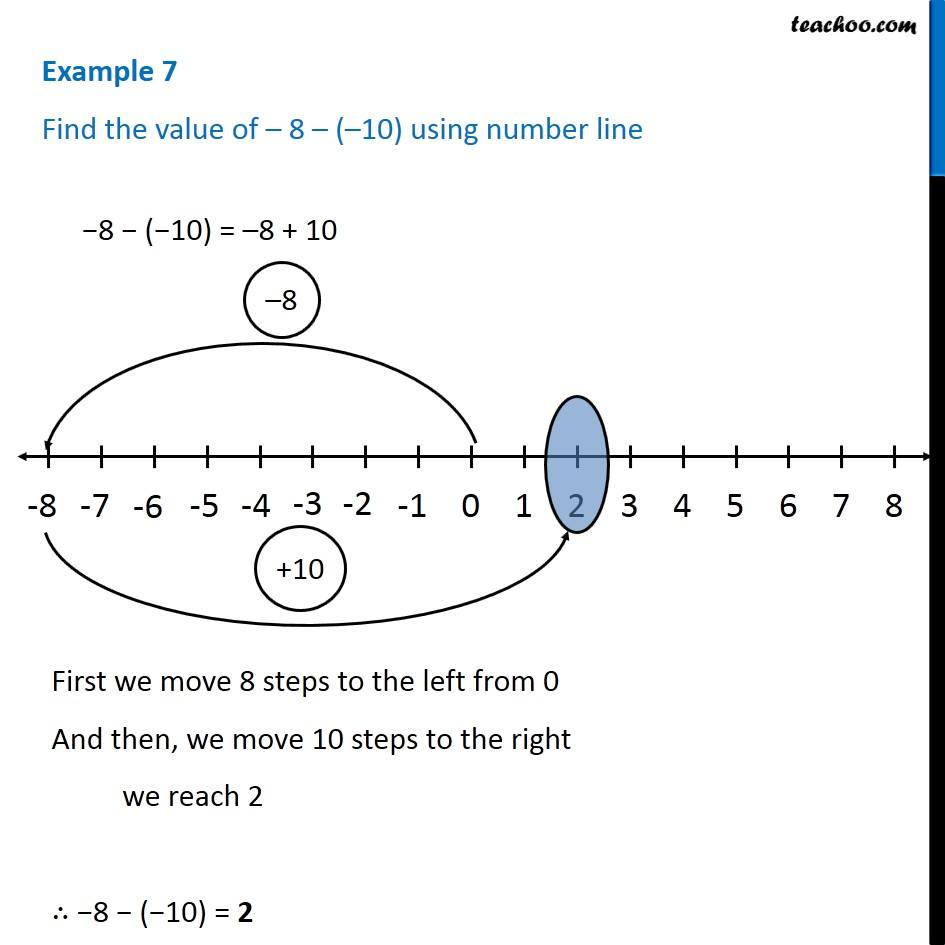1. Chapter 6 Class 6 Integers
2. Serial order wise
3. Examples

Transcript

Example 7 Find the value of – 8 – (–10) using number line −8 − (−10) = –8 + 10 First we move 8 steps to the left from 0 And then, we move 10 steps to the right we reach 2 ∴ −8 − (−10) = 2